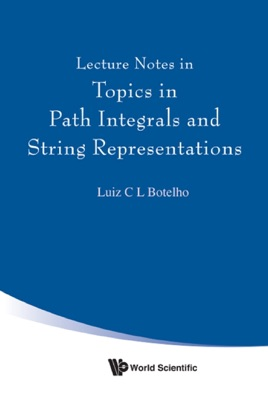• USD 38.99

## Descripción de editorial

Functional Integrals is a well-established method in mathematical physics, especially those mathematical methods used in modern non-perturbative quantum field theory and string theory. This book presents a unique, original and modern treatment of strings representations on Bosonic Quantum Chromodynamics and Bosonization theory on 2d Gauge Field Models, besides of rigorous mathematical studies on the analytical regularization scheme on Euclidean quantum field path integrals and stochastic quantum field theory. It follows an analytic approach based on Loop space techniques, functional determinant exact evaluations and exactly solubility of four dimensional QCD loop wave equations through Elfin Botelho fermionic extrinsic self avoiding string path integrals.
0Readership: Applied mathematicians, mathematical physicists and theoretical physicists.
Strings, Quantum Chromodynamics, Loop Spaces, Path Integrals0